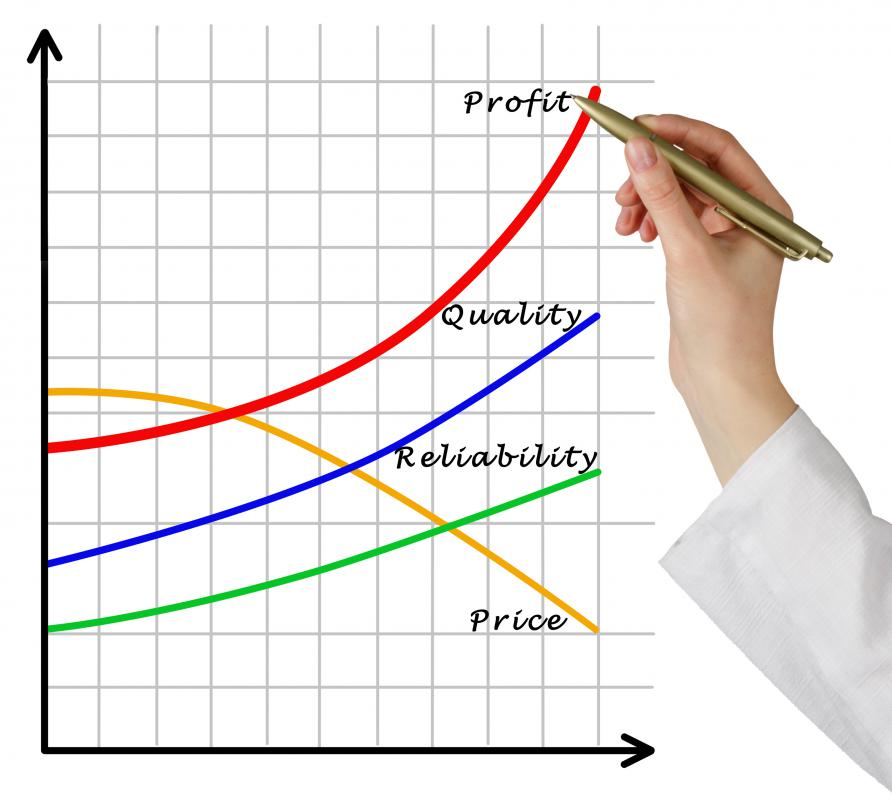# What Is an Average Profit Margin? (with pictures)

Helen Akers
Helen AkersBusinesses often calculate their average profit margin over a certain period of time.

An average profit margin is one that is observed over a certain time frame. It can also be calculated in production volume terms as the profit margin per unit. The total production and marketing costs are spread out equally over all units and subtracted from the total revenue. This figure is then divided by the total volume of produced units to arrive at the average profit margin per unit.An average profit margin can also be calculated in production volume terms as the profit margin per unit.

Companies may want to calculate an average profit margin over the past five years. In order to accomplish this, they would first calculate the profit margin for each year. For example, sales for year one would be subtracted from that year's expenses to arrive at an annual profit margin. This would be repeated for years two through five. All of the annual profit margins would then be added together and divided by five in order to determine the average profit margin.

Measuring an average profit margin against different business units and industry averages is also possible. The time frames for the profit margins that are being compared against each other should match. For instance, if the profit margins for soft drinks are being compared against snack foods for the previous two years, the calculations should include data that begins with the same month and ends with the same month.

Calculating an average profit margin per unit is a bit more detailed. It involves taking into consideration all of the costs that can be attributed towards the manufacture and sale of a particular product line. For example, advertising costs, promotional costs and manufacturing costs, including salaries and wages, would be added separately for soft drinks and snack foods. Joint advertising costs are typically divided equally between the product lines involved.

Once all of the costs are totaled and attributed towards a specific product line, they are divided by the number of units. For instance, the total production costs for soft drinks may equal \$100 US Dollars (USD). The total advertising costs may equal \$50 USD. With those costs, the total soft drinks produced might equal 200 units.

In this case, the average production costs per unit would be \$0.50 USD per unit. The average advertising costs would be \$0.25 USD per unit. If the soft drinks retail at \$1.50 USD per unit, the average profit margin per unit would total \$0.75 USD. For this example there is a positive profit margin, but negative margins or losses are possible.

## You might also Like## Discuss this Article

Forgot password?
###### Register:
•Businesses often calculate their average profit margin over a certain period of time.
•An average profit margin can also be calculated in production volume terms as the profit margin per unit.